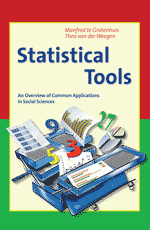23 Sep# R Sessions 33: Select (nested) observations with equal number of occurences

Recently, I was contacted with an question about R code. A befriended researcher was working with nested data, which was unbalanced. He was working with data in a ‘long’ format: all observations nested within the same group had the same identification number. But, the number of observations in each of the groups differed (hence: unbalanced data).

He asked me for a piece of code that creates a subset of the data that is balanced, i.e. all observations that are nested within equally sized groups. Or, as an alternative, all observations nested within groups with at least a minimum number of observations.

I solved it the quick and dirty way, and the solution involves creating additional variables, a new data.frame, and merging. It sure can be done much prettier, but it works.

So, I share it below:

``` id <- c("a", "b","b", "c","c","c", "d","d","d","d", "e","e","e") y <- c(3,4,3,2,4,5,6,5,6,7,5,4,3) df <- data.frame(id, y) # setting up original data.frame```

``` tab <- data.frame(id=names(table(df\$id)), fre=as.vector(table(df\$id))) # table of frequencies df.new <- merge(df, tab, by="id") # merging frequencies-variable ```

```subset(df.new, fre==3) # subsetting subset(df.new, fre>3) ```